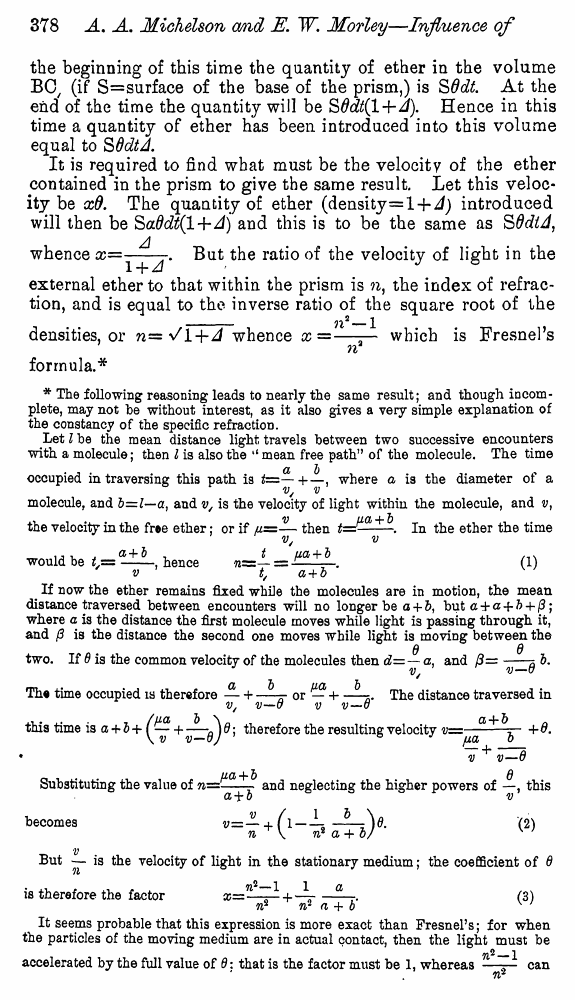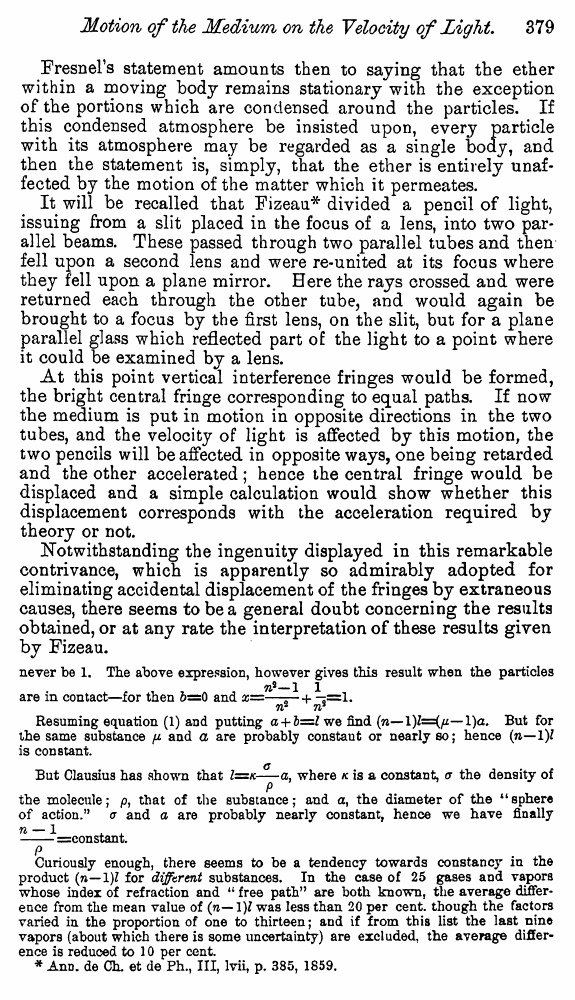﻿ Michelson A.A., Morley E. Influence of Motion of the Medium on the velocity of Light. // American Journal of Science, Ser. 3, 1886, vol. 31

# Michelson A.A., Morley E. Influence of Motion of the Medium on the velocity of Light. // American Journal of Science, Ser. 3, 1886, vol. 31

В начало   Другие форматы   <<<     Страница 378   >>>377  378 379  380  381  382  383  384  385  386 the beginning of this time the quantity of ether in the volume B0/ (if S=surface of the base of the prism,) is SOdt At the end of the time the quantity will be SOdtil+A). Hence in this time a quantity of ether has been introduced ioto this volume equal to S Odt A. It is required to find what must be the velocity of the ether contained in the prism to give the same result. Let this velocity be xO. The quantity of ether (density=l-J- A) introduced will then be SaOdt(l+A) and this is to be the same as SOdiA, whence X==Y^J' ^ut rat*° ve'oc^y in the external ether to that within the prism is n, the index of refraction, and is equal to the inverse ratio of the square root of the __ji2_1 densities, or n='/l-hA whence x =—— which is Fresnel’s n formula.* * The following reasoning leads to nearly the same result; and though incomplete, may not he without interest, as it also gives a very simple explanation of the constancy of the specific refraction. Let I he the mean distance light travels between two successive encounters with a molecule; then I is also the ‘‘ mean free path” of the molecule. The time occupied in traversing this path is fc=— +—, where a is the diameter of a molecule, and b=l—a, and v/ is the velocity of light within the molecule, and v, the velocity in the free ether: or if ll=— then #=jtza + -. ln the ether the time v/ v would be /,= , hence 71= — = + ^ . (1) ' v t, a + b If now the ether remains fixed while the molecules are in motion, the mean distance traversed between encounters will no longer be a + &, but a + a + b 4- (3; where a is the distance the first molecule moves while light is passing through it, and /? is the distance the second one moves while light is moving between the q d two. If 6 is the common velocity of the molecules then d= — a, and 3=-3 b. The time occupied is therefore — + —r or — H--s<. The distance traversed in v/ v—u v v—o , . . /UOL b \ CL + b 0 this time is a + b + f J Q; therefore the resulting velocity j • v v—B ua + b 6 Substituting the value of n=-r and neglecting the higher powers of —, this a + o ^ becomes v~— + (1—(2) n^\ n* a+ b/ w v But is the velocity of light in the stationary medium; the coefficient of 6 is therefore the factor --(3) n* w a + b w It seems probable that this expression is more exact than Fresnel’s; for when the particles of the moving medium are in actual contact, then the light must be ^2_1 accelerated by the full value of 0: that is the factor must be 1, whereas —— can Fresnel’s statement amounts then to saying that the ether within a moving body remains stationary with the exception of the portions which are condensed around the particles. If this condensed atmosphere be insisted upon, every particle with its atmosphere may be regarded as a single body, and then the statement is, simply, that the ether is entirely unaffected by the motion of the matter which it permeates. It will be recalled that Fizeau* divided a pencil of light, issuing from a slit placed in the focus of a lens, into two parallel beams. These passed through two parallel tubes and then fell upon a second lens and were re-united at its focus where they fell upon a plane mirror. Here the rays crossed and were returned each through the other tube, and would again be brought to a focus by the first lens, on the slit, but for a plane parallel glass which reflected part o£ the light to a point where it could be examined by a lens. At this point vertical interference fringes would be formed, the bright central fringe corresponding to equal paths. If now the medium is put in motion in opposite directions in the two tubes, and the velocity of light is affected by this motion, the two pencils will be affected in opposite ways, one being retarded and the other accelerated; hence the central fringe would be displaced and a simple calculation would show whether this displacement corresponds with the acceleration required by theory or not. Notwithstanding the ingenuity displayed in this remarkable contrivance, which is apparently so admirably adopted for eliminating accidental displacement of the fringes by extraneous causes, there seems to be a general doubt concerning the results obtained, or at any rate the interpretation of these results given by Fizeau. never be 1. The above expression, however gives this result when the particles 7l2—1 1 are in contact—for then 5=0 and x=—— + -*=1. 71 71 Resuming equation (1) and putting a + b=l we find (w— l)a. But for the same substance p and a are probably constant or nearly so; hence (n—1)1 is constant. But Clausius has shown that Z=/c-^-a, where k is a constant, a the density of 9 the molecule; p, that of the substance; and a, the diameter of the “sphere of action.” a and a are probably nearly constant, hence we have finally n — 1 . . -=constant. P . ^ Curiously enough, there seems to be a tendency towards constancy in the product (ti—1)? for different substances. In the case of 25 gases and vapors whose index of refraction and “ free path” are both known, the average difference from the mean value of (n— 1)J was less than 20 per cent, though the factors varied in the proportion of one to thirteen; and if from this list the last nine vapors (about which there is some uncertainty) are excluded, the average difference is reduced to 10 per cent. * AnD. de Ch. et de Ph., Ill, lvii, p. 385, 1S59.